# 模型结构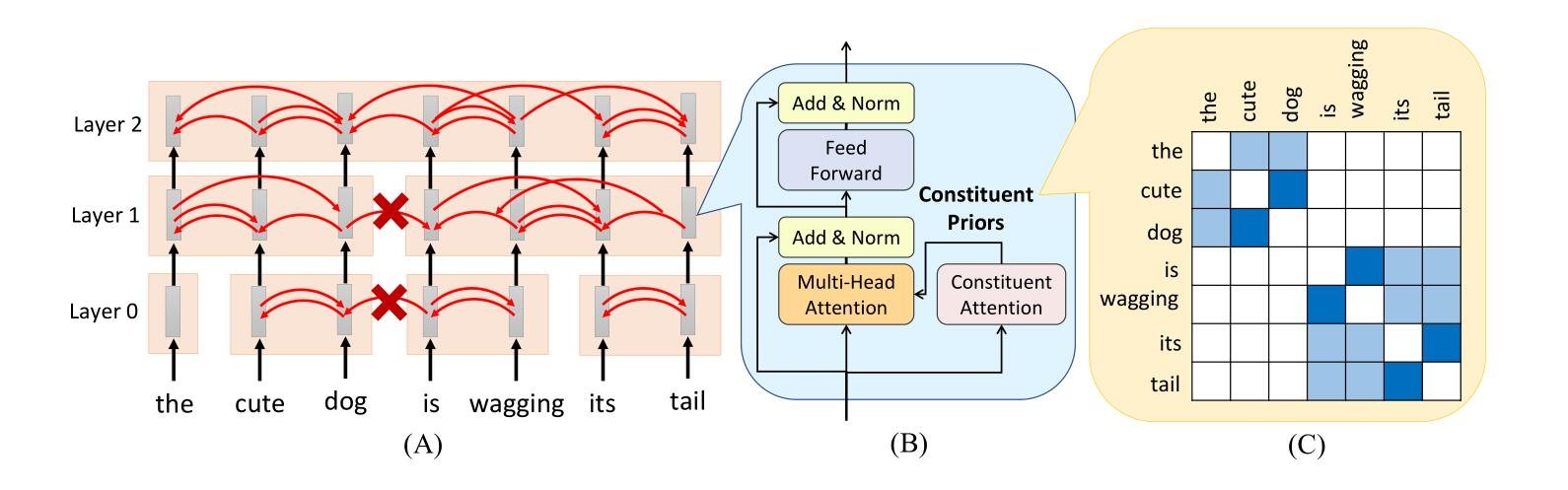$$E = \text{softmax}(\frac{QK^{\top}}{d})$$

$$E = C \odot \text{softmax}(\frac{QK^{\top}}{d})$$

$$C_{i, j} = \prod_{k=i}^{j-1}{a_k}$$

$$s_{i, i+1} = \frac{q_i \cdot k_{i+1}}{d}$$

$$p_{i,i+1}, p_{i, i-1} = \text{softmax}(s_{i,i+1}, s_{i, i-1})$$

$$\hat{a}_i = \sqrt{p_{i, i+1} \times p_{i+1, i}}$$

$$a_i^l = a_i^{l-1} + (1 - a_i^{l-1})\hat{a}_i^l$$

# 无监督句法分析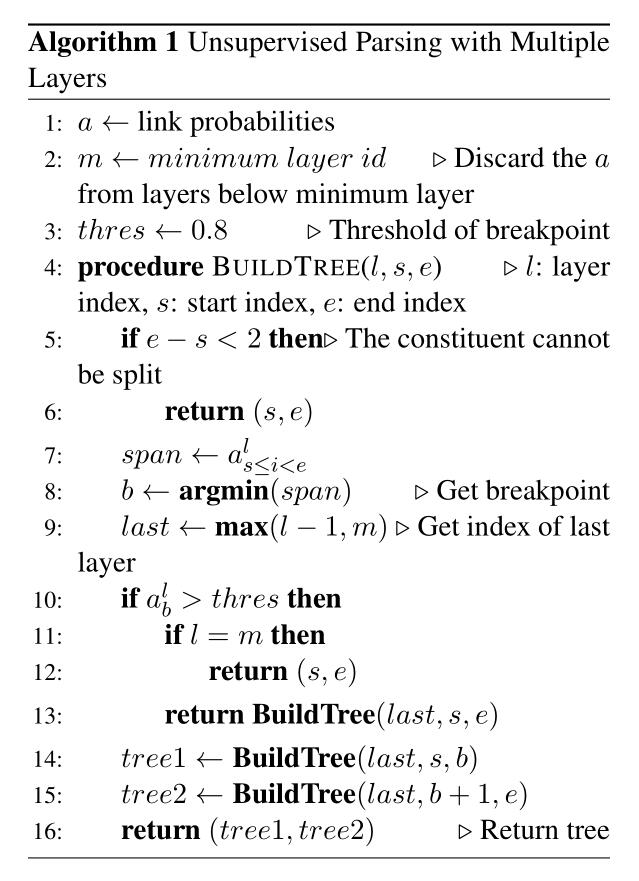# 实验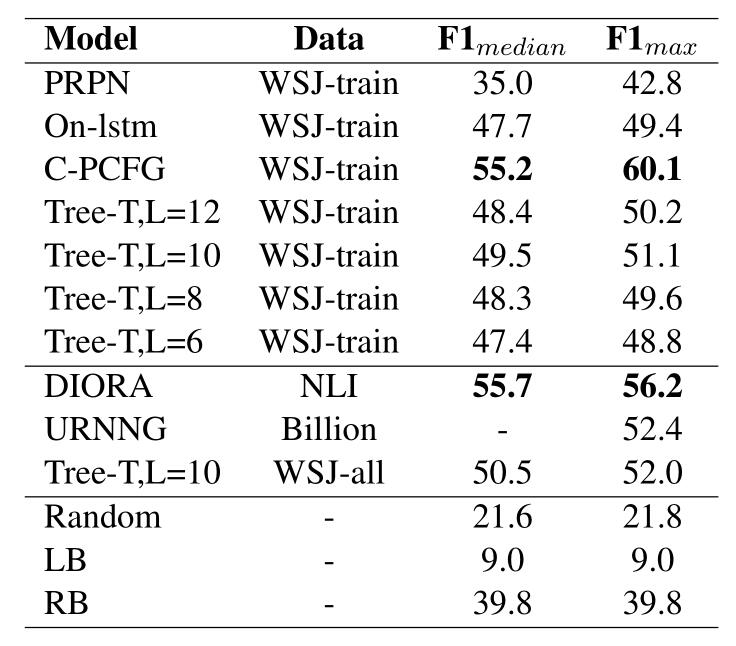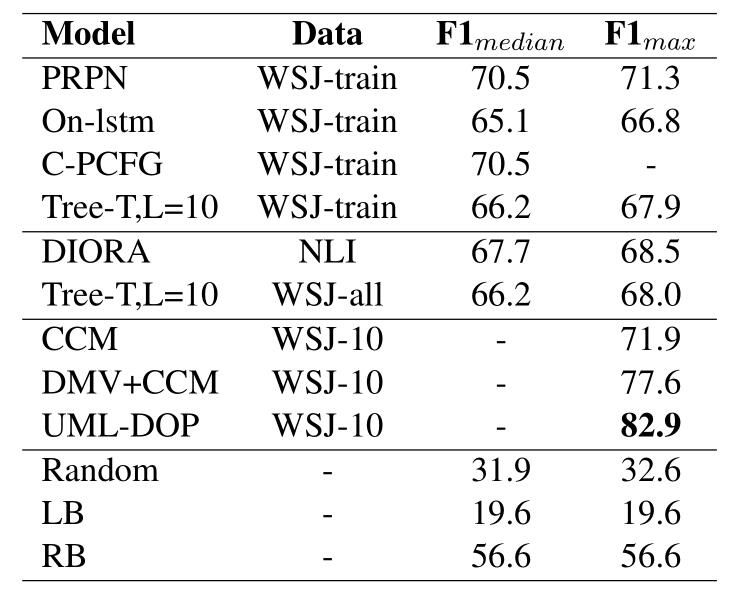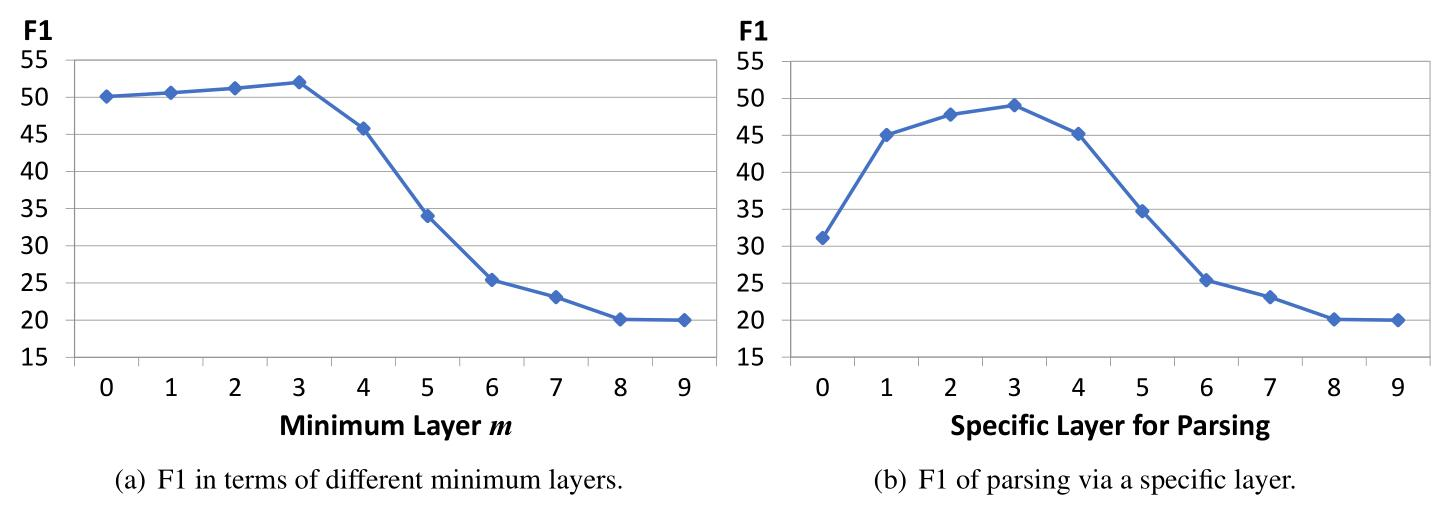# 结论

转载规则

《论文赏析[EMNLP19]如何在Transformer中融入句法树信息？这里给出了一种解决方案》 韦阳 采用 知识共享署名 4.0 国际许可协议 进行许可。
上一篇每日算法系列【LeetCode 689】三个无重叠子数组的最大和

2020-01-07论文赏析[TACL19]生成模型还在用自左向右的顺序？这篇论文教你如何自动推测最佳生成顺序

2020-01-05
目录